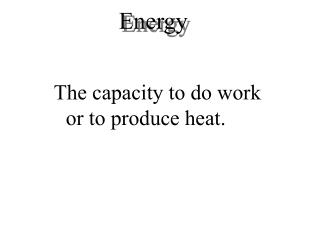DownloadDownload PresentationEnergy

# Energy

Télécharger la présentation## Energy

- - - - - - - - - - - - - - - - - - - - - - - - - - - E N D - - - - - - - - - - - - - - - - - - - - - - - - - - -
##### Presentation Transcript

1. Energy The capacity to do work or to produce heat.

2. System and Surroundings System: That on which we focus attention Surroundings: Everything else in the universe Universe = System + Surroundings

3. Figure 6.9 Components of internal energy (E)

4. Components of Internal Energy Contributions to the kinetic energy: • The molecule moving through space, Ek(translation) • The molecule rotating, Ek(rotation) • The bound atoms vibrating, Ek(vibration) • The electrons moving within each atom, Ek(electron) Contributions to the potential energy: • Forces between the bound atoms vibrating, Ep(vibration) • Forces between nucleus and electrons and between electrons in each atom, Ep(atom) • Forces between the protons and neutrons in each nucleus, Ep(nuclei) • Forces between nuclei and shared electron pair in each bond, Ep(bond)

5. Exo and Endothermic Heat exchange accompanies chemical reactions. Exothermic: Heat flows out of the system (to the surroundings). Endothermic: Heat flows into the system (from the surroundings).

6. Exothermic versus endothermic.

7. Figure 6.3: The energy diagram for the reaction of nitrogen and oxygen to form nitric oxide. This is an endothermic process: Heat {equal in magnitude to ∆(PE)} flows into the system from the surroundings.

8. Figure 6.2: The combustion of methane releases the quantity of energy change ∆(PE) to the surroundings via heat flow. This is an exothermic process.

9. The Two Types of Energy Potential:due to position or composition - can be converted to work Kinetic:due to motion of the object KE = 1/2 mv2 (m = mass, v = velocity)

10. Work work = force distance since pressure = force / area, work = pressure  volume wsystem = PV

11. Figure 6.4: (a) The piston, moving a distance ∆h against a pressure P, does work on the surroundings. (b) Since the volume of a cylinder is the area of the base times its height, the change in volume of the gas is given by ∆h x A = V.

12. Law of Conservation of Energy Energy can be converted from one form to another but can neither be created nor destroyed. (Euniverse is constant)

13. First Law E = q+ w E = change in system’s internal energy q = heat w = work

14. Figure 6.2 Energy diagrams for the transfer of internal energy (E) between a system and its surroundings. DE = Efinal - Einitial = Eproducts - Ereactants

15. DE = q + w Figure 6.3 A system transferring energy as heat only.

16. Figure 6.4 A system losing energy as work only.

17. State Function Depends only on the present state of the system - not how it arrived there. It is independent of pathway.

18. Enthalpy Enthalpy = H = E + PV E = HPV H = E + PV At constant pressure, qP = E + PV, where qP = H at constant pressure H = energy flow as heat (at constant pressure)

19. Hess’s Law Reactants  Products The change in enthalpy is the samewhether the reaction takes place in one step or a series of steps.

20. Figure 6.8: Pathway for combustion of methane.

21. Figure 6.9: A schematic diagram of the energy changes for the reaction CH4(g) + 2O2(g) CO2(g) + 2H2O(l).

22. Figure 6.7: The principle of Hess's law. The same change in enthalpy occurs when nitrogen and oxygen react to form nitrogen dioxide, regardless of whether the reaction occurs in one (red) or two (blue) steps.

23. Calculations via Hess’s Law 1. If a reaction is reversed, H is also reversed. N2(g) + O2(g)  2NO(g) H = 180 kJ 2NO(g)  N2(g) + O2(g) H = 180 kJ 2. If the coefficients of a reaction are multiplied by an integer, H is multiplied by that same integer. 6NO(g) 3N2(g) + 3O2(g) H = 540 kJ

24. Standard States Compound • For a gas, pressure is exactly 1 atmosphere. • For a solution, concentration is exactly 1 molar. • Pure substance (liquid or solid), it is the pure liquid or solid. Element • The form [N2(g), K(s)] in which it exists at 1 atm and 25°C.

25. Change in Enthalpy Can be calculated from enthalpies of formation of reactants and products. Hrxn° = npHf(products) nrHf(reactants)